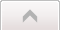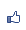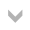Contents ...
udn網路城邦

2021/10/16 03:59

「奈」是 nano，nano 就是「奈」。

10**6 = 2**20 = mega（百萬）

10**-6 = 2**-20 = micro

10**9 = 2**30 = giga（十億）

10**-9 = 2**-30 = nano（「奈」）

10**12 = 2**40 = tera（兆）

10**-12 = 2**-40 = pico